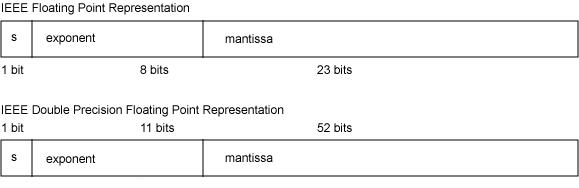# 1.浮点数的存储格式

IEEE754 标准中规定 float 单精度浮点数在机器中表示用 1 位表示数字的符号，用 8 位表示指数，用 23 位表示尾数，即小数部分。对于 double 双精度浮点数，用 1 位表示符号，用 11 位表示指数，52 位表示尾数，其中指数域称为阶码。IEEE754 浮点数的格式如下图所示。# 2.浮点数的规格化

## 2.1单精度浮点数真值

IEEE754标准中，一个规格化32位的浮点数x的真值表示为：
$x=(-1)^S\times(1.M)\times2^e$
$e=E-127$

## 2.2双精度浮点数真值

64位的浮点数中符号为1位，阶码域为11位，尾数域为52位，指数偏移值是1023。因此规格化的64位浮点数x的真值是：
$x=(-1)^S\times(1.M)\times2^e$
$e=E-1023$

# 3.移码

（1）原码符号位为1不变，整数的每一位二进制数位求反得反码；
（2）反码符号位为1不变，反码数值位最低位加1得补码。

# 4.浮点数的具体表示

## 4.1十进制到机器码

（1）0.5
$0.5=(0.1)_2$，符号位S为0，指数为$e=-1$，规格化后尾数为1.0。

$M=[000\ 0000\ 0000\ 0000\ 0000\ 0000]_2$

$E=[-1]_移-1=[0111\ 1111]_2-1=[0111\ 1110]_2$

$0.5=[0011\ 1111\ 0000\ 0000\ 0000\ 0000\ 0000\ 0000]_2$

（2）1.5
$1.5=[1.1]_2$，符号位为0，指数$e=0$，规格化后尾数为1.1。

$M=[100\ 0000\ 0000\ 0000\ 0000\ 0000]_2$

$E=_移-1=[1000 0000]_2-1=[0111 1111]_2$

$1.5=[0011\ 1111\ 1100\ 0000\ 0000\ 0000\ 0000\ 0000]_2$

（3）-12.5
$-12.5=[-1100.1]_2$，符号位S为1，指数e为3，规格化后尾数为1.1001，

$M=[100\ 1000\ 0000\ 0000\ 0000\ 0000]_2$

$E=_移-1=[1000\ 0011]_2-1=[1000\ 0010]_2$

$-12.5=[1100\ 0001\ 0100\ 1000\ 0000\ 0000\ 0000\ 0000]_2$

#include <iostream>
using namespace std;

int main()
{
float a=0.5;
float b=1.5;
float c=-12.5;

unsigned int* pa=NULL;
pa=(unsigned int*)&a;
unsigned int* pb=NULL;
pb=(unsigned int*)&b;
unsigned int* pc=NULL;
pc=(unsigned int*)&c;

cout<<hex<<"a=0x"<<*pa<<endl;
cout<<hex<<"b=0x"<<*pb<<endl;
cout<<hex<<"c=0x"<<*pc<<endl;

return 0;
}


a=0x3f000000
b=0x3fc00000
c=0xc1480000


## 4.2机器码到十进制

（1）若浮点数x的IEEE754标准存储格式为0x41360000，那么其浮点数的十进制数值的推演过程如下：

$0x41360000=[0\ 10000010\ 011\ 0110\ 0000\ 0000\ 0000\ 0000]$

$x=(-1)^S\times1.M\times2^e=+(1.011011)\times2^3=+1011.011=(11.375)_{10}$

#include <iostream>
using namespace std;

int main()
{
unsigned int hex=0x41360000;
float* fp=(float*)&hex;
cout<<"x="<<*fp<<endl;
return 0;
}


x=11.375


# 5.浮点数的几种特殊情况

（1）0的表示

-0的机器码为：1 00000000 000 0000 0000 0000 0000 0000。

$x=(-1)^S\times(1.M)\times2^e$
$e=E-127$

（2）$+\infty $和$-\infty$的表示

（3）NaN（Not a Number）

# 6.浮点数的精度和数值范围

## 6.1浮点数的数值范围

（1）最大正数

$PosMax=(-1)^S\times1.M\times2^e=+(1.111 1111 1111 1111 1111 1111)\times2^{127}\approx3.402823e+38$

（2）最小正数

$PosMin=(-1)^S\times1.M\times2^e=+(1.0)\times2^{-126} \approx1.175494e-38$

（3）最大负数

$NegMax=(-1)^S\times1.M\times2^e=-(1.0)\times2^{-126} \approx-1.175494e-38$

（4）最小负数

$NegMin=(-1)^S\times1.M\times2^e=+(1.111 1111 1111 1111 1111 1111)\times2^{127}=-3.402823e+38$

## 6.2浮点数的精度

64位双精度浮点数的尾数域52位，因$2^{52}-1=4,503,599,627,370,495$，所以双精度浮点数的十进制的精度最高为16位，绝对保证的为15位，所以double的十进制的精度为15~16位。

# 参考文献

移码.百度百科
关于IEEE754标准浮点数阶码的移码.百度知道
计算机组成原理第四版[M].白中英.科学出版社:P16-P30
浮点数.维基百科

©️2019 CSDN 皮肤主题: 编程工作室 设计师: CSDN官方博客